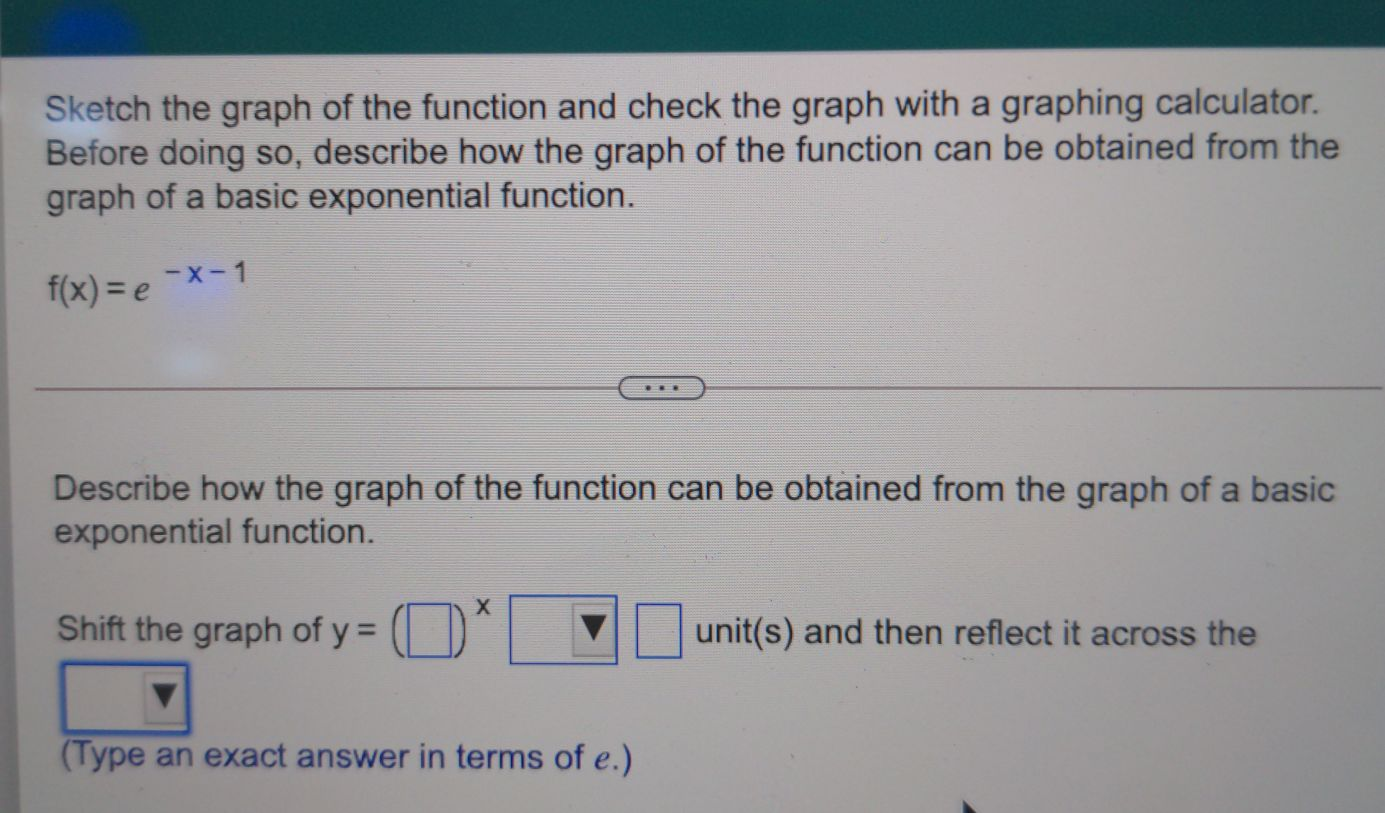### Still have math questions?

Algebra
QuestionSketch the graph of the function and check the graph with a graphing calculator. Before doing so, describe how the graph of the function can be obtained from the graph of a basic exponential function. $$f ( x ) = e ^ { - x - 1 }$$ Describe how the graph of the function can be obtained from the graph of a basic exponential function. Shift the graph of $$y = ( \square ) ^ { x } \square$$ unit(s) and then reflect it across the

$$\square \square$$

shift the graph of y=e$$^x$$ right 1 unit and then reflect it across the y-axis